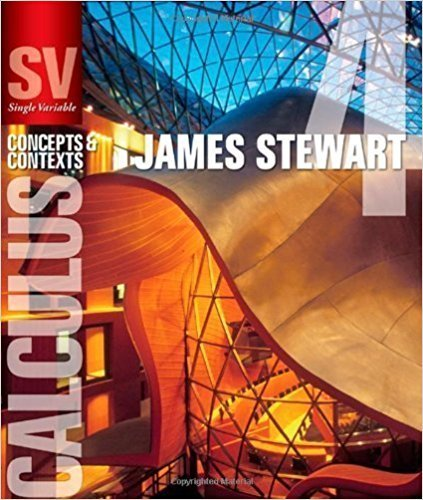×
×

# Solutions for Chapter 5.8: INTEGRATION USING TABLES AND COMPUTER ALGEBRA SYSTEMS## Full solutions for Single Variable Calculus: Concepts and Contexts (Stewart's Calculus Series) | 4th Edition

ISBN: 9780495559726Solutions for Chapter 5.8: INTEGRATION USING TABLES AND COMPUTER ALGEBRA SYSTEMS

Solutions for Chapter 5.8
4 5 0 414 Reviews
30
0
##### ISBN: 9780495559726

This expansive textbook survival guide covers the following chapters and their solutions. Chapter 5.8: INTEGRATION USING TABLES AND COMPUTER ALGEBRA SYSTEMS includes 34 full step-by-step solutions. Since 34 problems in chapter 5.8: INTEGRATION USING TABLES AND COMPUTER ALGEBRA SYSTEMS have been answered, more than 20936 students have viewed full step-by-step solutions from this chapter. Single Variable Calculus: Concepts and Contexts (Stewart's Calculus Series) was written by and is associated to the ISBN: 9780495559726. This textbook survival guide was created for the textbook: Single Variable Calculus: Concepts and Contexts (Stewart's Calculus Series), edition: 4.

Key Calculus Terms and definitions covered in this textbook
• Absolute value of a complex number

The absolute value of the complex number z = a + b is given by ?a2+b2; also, the length of the segment from the origin to z in the complex plane.

• Arccosecant function

See Inverse cosecant function.

• Average velocity

The change in position divided by the change in time.

• Cofunction identity

An identity that relates the sine, secant, or tangent to the cosine, cosecant, or cotangent, respectively

• Inequality symbol or

<,>,<,>.

• Inferential statistics

Using the science of statistics to make inferences about the parameters in a population from a sample.

See Polynomial function in x.

• Length of a vector

See Magnitude of a vector.

• Limit

limx:aƒ1x2 = L means that ƒ(x) gets arbitrarily close to L as x gets arbitrarily close (but not equal) to a

• Multiplication principle of counting

A principle used to find the number of ways an event can occur.

• Normal curve

The graph of ƒ(x) = e-x2/2

• Open interval

An interval that does not include its endpoints.

• Parameter interval

See Parametric equations.

• Principal nth root

If bn = a, then b is an nth root of a. If bn = a and a and b have the same sign, b is the principal nth root of a (see Radical), p. 508.

• Rational numbers

Numbers that can be written as a/b, where a and b are integers, and b ? 0.

• Real zeros

Zeros of a function that are real numbers.

• Recursively defined sequence

A sequence defined by giving the first term (or the first few terms) along with a procedure for finding the subsequent terms.

• Sum of a finite arithmetic series

Sn = na a1 + a2 2 b = n 2 32a1 + 1n - 12d4,

• Vertex of a parabola

The point of intersection of a parabola and its line of symmetry.

• y-intercept

A point that lies on both the graph and the y-axis.

×